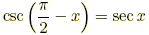# Simplify cofunction expression

• synergix

## Homework Statement

csc(pi/2-x)/cos(x+pi/2) + cot(pi/2-x)

## The Attempt at a Solution

1/cosx/-sinx + sinx/cosx

-1/sinxcosx+ sinx/cosx(sinx/sinx)

(sin2x - 1) / sinxcosx

-cos2x/sinxcosx

-cosx/sinx

-cotx

is this right the answer key says it is cosx but that could be wrong I have done this a couple times and gotten the same answer

I don't think it can be the same as cosx because if you put x=0, it doesn't work.

csc(pi/2-x)/cos(x+pi/2) + cot(pi/2-x)
1/cosx/-sinx + sinx/cosx
I'm assuming you mean (1/cosx)/-sinx in the first fraction. Anyway, this is not correct.

01

(1)(2)cos(x+pi/2) = - sinx

(1)/(2)=

secx/-sinx=

(1/cosx)/-sinx